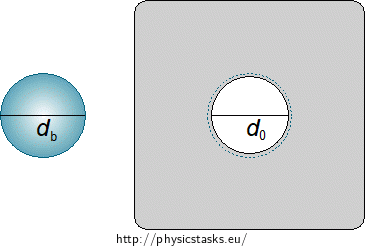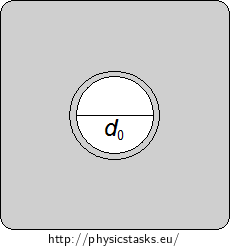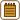## Change in the Metal Plate's Size during Heating

There is an aluminium plate measuring 10 cm x 10 cm and a round hole, whose diameter is 36 mm, in the centre of the plate. By how many degrees do we have to warm the plate up so that a ball with a diameter of 36.1 mm could pass through the hole?

• #### Notation

 d0 = 36.0 mm = 3.60·10−2 m diameter of the round hole before heating l0 circumference of the round hole before heating db = 36.1 mm = 3.61·10−2 m diameter of the ball lb circumference of the smallest hole through which the ball could pass

From the Tables:

 α = 2.4·10−5 K−1 coefficient of linear thermal expansion of aluminium
• #### Analysis

The circumference of the hole in the plate is smaller than the circumference of the ball’s projection into the plate’s plane (hereinafter the circumference of the ball). Due to thermal expansion, the dimensions of the plate and the hole in the middle will get larger, if we heat the plate up. The ball will then be able to pass through the hole.

To solve the task, we have to know the relation for the change in object length with regard to linear thermal expansion. We use this relation to calculate changes in lengths of objects whose one dimension is considerably larger than the other two (e.g. rods or wires). We can imagine that the metal consists of lots of thin metal strips. Then we can use the relation for linear thermal expansion to calculate its enlargement cased by heating.

Fig. 1: The circumference of the ball is represented by a dashed line around the hole in the plate.The circumference of the hole must be enlarged at least to the ball’s circumference. So we write the relation for the enlarged circumference of the hole after heating and substitute the circumference of the ball for it. Therefrom, we express the required temperature change.

• #### Hint

Imagine the plate being “cut” into thin concentric rings whose centres lie in the centre of the hole. Express the change in the circumference of such a ring after increasing its temperature by Δt.

• #### SolutionWe calculate the circumference of the round hole of the diameter d0 before the heating from:

$l_{\mathrm{0}} = πd_{\mathrm{0}}.\tag{1}$

If the temperature of the aluminium plate is increased by Δt, the round hole will enlarge and we can apply the following relation for its circumference l:

$l = l_{\mathrm{0}}(1+αΔt).\tag{2}$

The ball’s diameter is db. Therefore, the diameter of the hole through which the ball would pass must be at least equal to db. The circumference of such a hole lb can be calculated from:

$l_{\mathrm{b}} = πd_{\mathrm{b}}.\tag{3}$

In order for the ball to pass through the hole, its circumference after heating must be at least equal to lb. So we substitute l for the circumference of the ball lb in relation (2):

$l_{\mathrm{b}} = l_{\mathrm{0}}(1+αΔt).$

Now we substitute for relation (1) and (3) in relation (2):

$πd_{\mathrm{b}} = πd_{\mathrm{0}}(1+αΔt),$

Then we adjust the relation and express Δt from it:

$\frac{d_{\mathrm{b}}}{d_{\mathrm{0}}}−1 = αΔt$ $Δt = \frac{d_{\mathrm{b}}−d_{\mathrm{0}}}{α·d_{\mathrm{0}}}.\tag{4}$

Then we substitute for the values from the task assignment in relation (4) and calculate, by how many degrees we have to heat the plate up:

$Δt = \frac{3.61·10^{−2}−3.60·10^{−2}}{2.4·10^{−5}·3.60·10^{−2}} °\mathrm{C}$ $Δt \dot{=} 115.7 °\mathrm{C}.$